# Pyramid calculation

Description and formulas for the calculation of pyramids

## Pyramid Definition

• The base of a pyramid is a polygon with at least three edges.

• The number of edges of the base determines the number of slopes of the pyramid.

• The sides of a pyramid are triangular. They run inwards from the bases and meet at the top.

## Formulas for the calculation of pyramids

The following formulas refer to the calculation of a right quadrangular pyramid

#### Calculate base side length $$a$$ of a pyramid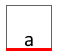$$\displaystyle a=\sqrt{\frac{P}{4}}$$

#### Calculate radius to a side $$r_s$$ of a pyramid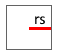$$\displaystyle r_s=\sqrt{\frac{A}{2}}$$

#### Calculate radius to a vertex $$r_v$$ of a pyramid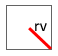$$\displaystyle r_v=\sqrt{(a/2)^2+{r_s}^2}$$

#### Calculate perimeter of the base $$P$$ of a pyramid$$\displaystyle P=4·a$$

#### Calculate base area $$A$$ of a pyramid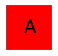$$\displaystyle A=a^2$$

#### Calculate height $$h$$ of a pyramid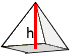$$\displaystyle h=\frac{3·V}{A}$$

$$\displaystyle h=\sqrt{m^2-{r_s}^2}$$

#### Calculate slant height $$m$$ of a pyramid$$\displaystyle m=\sqrt{h^2+{r_s}^2}$$

#### Calculate edge length $$k$$ of a pyramid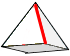$$\displaystyle k=\sqrt{m^2+(a^2/4)}$$

#### Calculate area of a slope $$M_1$$ of a pyramid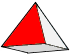$$\displaystyle M_1=\frac{m · a}{2}$$

#### Calculate lateral area $$M$$ of a pyramid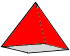$$\displaystyle M=\frac{m · P}{2}$$

#### Calculate volumen $$V$$ of a pyramid$$\displaystyle V=\frac{A · h}{3}$$